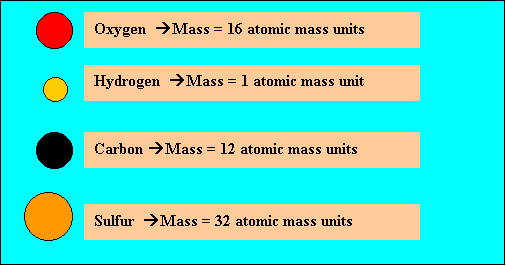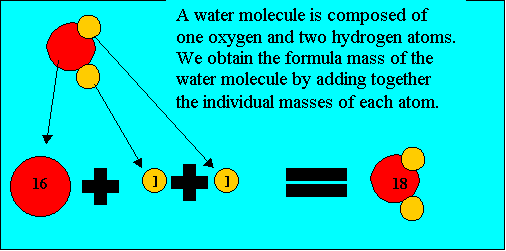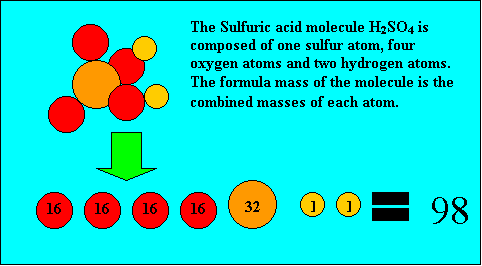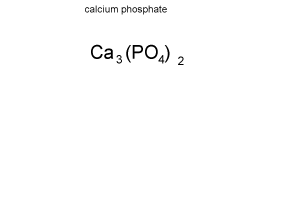Formula mass Molecules have a molecular (formula) mass, equivalent to the combined mass of all the atoms that make up the molecule. The atomic masses of elements are found in text books or the periodic table and are expressed in atomic mass units. For example, take oxygen, hydrogen, carbon and sulfur. Their atomic masses are shown on the right.Before you can calculate the formula mass of a compound it is important you know how to read a chemical formula. For example calculate the formula mass of calcium phosphate (Ca3(PO4)2) Step 1 Calculate how many atoms of each element are present in the formula of the compound. The animation on the left breaks down the formula to reveal : - 3 atoms of calcium - 2 atoms of phosphorus - 8 atoms of oxygen. Step 2 Find the atomic mass of each element present Ca = 40.1 atomic mass units P = 31.0 atomic mass units O = 16.0 atomic mass units Step 3 Multiply the atomic mass of each element by the number of atoms present and sum to get the total. Formula mass = 3 X 40.1 + 2 X 31.0 + 8 X 16.0 = 310.3 atomic mass units Try some exercises in calculating molecular (formula) mass.